Next: Hypothesis test (discrete case) Up: Conditional probability and Bayes' Previous: Conventional use of Bayes'   Contents

## Bayesian statistics: learning by experience

The advantage of the Bayesian approach (leaving aside the little philosophical detail'' of trying to define what probability is) is that one may talk about the probability of any kind of event, as already emphasized. Moreover, the procedure of updating the probability with increasing information is very similar to that followed by the mental processes of rational people. Let us consider a few examples of Bayesian use'' of Bayes' theorem.
Example 1:
Imagine some persons listening to a common friend having a phone conversation with an unknown person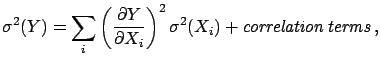, and who are trying to guess whois. Depending on the knowledge they have about the friend, on the language spoken, on the tone of voice, on the subject of conversation, etc., they will attribute some probability to several possible persons. As the conversation goes on they begin to consider some possible candidates for, discarding others, then hesitating perhaps only between a couple of possibilities, until the state of information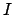is such that they are practically sure of the identity of. This experience has happened to most of us, and it is not difficult to recognize the Bayesian scheme: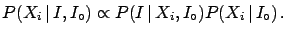(3.19)

We have put the initial state of information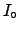explicitly in () to remind us that likelihoods and initial probabilities depend on it. If we know nothing about the person, the final probabilities will be very vague, i.e. for many personsthe probability will be different from zero, without necessarily favouring any particular person.
Example 2:
A person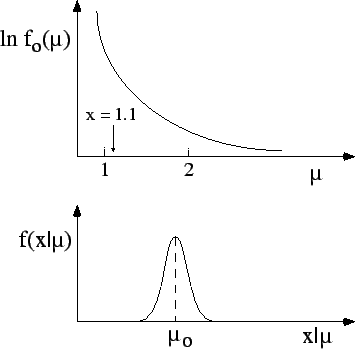meets an old friendin a pub.proposes that the drinks should be payed for by whichever of the two extracts the card of lower value from a pack (according to some rule which is of no interest to us).accepts andwins. This situation happens again in the following days and it is alwayswho has to pay. What is the probability thathas become a cheat, as the number of consecutive wins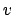increases?

The two hypotheses are: cheat (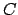) and honest (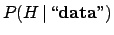).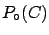is low becauseis an old friend'', but certainly not zero: let us assume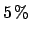. To make the problem simpler let us make the approximation that a cheat always wins (not very clever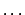):. The probability of winning if he is honest is, instead, given by the rules of probability assuming that the chance of winning at each trial is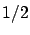(why not?", we shall come back to this point later):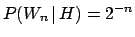. The result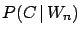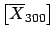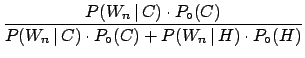(3.20)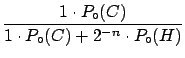(3.21)

is shown in the following table.(%) (%) 0 5.0 95.0 1 9.5 90.5 2 17.4 82.6 3 29.4 70.6 4 45.7 54.3 5 62.7 37.3 6 77.1 22.9Naturally, ascontinues to win the suspicion ofincreases. It is important to make two remarks.
• The answer is always probabilistic.can never reach absolute certainty thatis a cheat, unless he catchescheating, orconfesses to having cheated. This is coherent with the fact that we are dealing with random events and with the fact that any sequence of outcomes has the same probability (although there is only one possibility overin whichis always luckier). Making use of,can make a decision about the next action to take:
• continue the game, with probabilityof losing with certainty the next time too;
• refuse to play further, with probabilityof offending the innocent friend.
• Ifthe final probability will always remain zero: iffully trusts, then he has just to record the occurrence of a rare event whenbecomes large.

To better follow the process of updating the probability when new experimental data become available, according to the Bayesian scheme

the final probability of the present inference is the initial probability of the next one''.
Let us call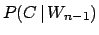the probability assigned after the previous win. The iterative application of the Bayes formula yields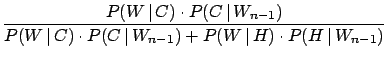(3.22)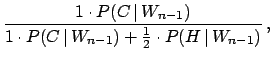(3.23)

where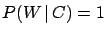and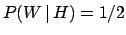are the probabilities of each win. The interesting result is that exactly the same values ofof () are obtained (try to believe it!).

It is also instructive to see the dependence of the final probability on the initial probabilities, for a given number of wins.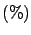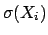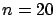24 91 99.7 99.9963 98 99.94 99.998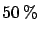97 99.90 99.997 99.9999

As the number of experimental observations increases the conclusions no longer depend, practically, on the initial assumptions. This is a crucial point in the Bayesian scheme and it will be discussed in more detail later.Next: Hypothesis test (discrete case) Up: Conditional probability and Bayes' Previous: Conventional use of Bayes'   Contents
Giulio D'Agostini 2003-05-15